﻿

### Some bounds for the genus of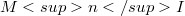$Mn I$

#### Abstract

Starting from a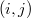$(i,j)$-symmetric crystallization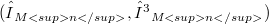$(Γ_{Mn},γ_{Mn})$ of a closed n-manifolds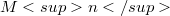$Mn$, we give an algorithm to build a crystallization(Error rendering LaTeX formula) OF$Mn I$. This algorithm allows to give a formula for the calculation of the regular genus of(Error rendering LaTeX formula), in the cases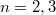$n=2,3$, and some bounds for the genus of the product-manifolds represented.

DOI Code: 10.1285/i15900932v18n2p175

Keywords: Product of manifolds for I; Crystallizations; Regular genus

Classification: 57N12; 57N13; 57Q15; 57M40

Full Text: PDF